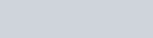# Derive an expression for potential energy of a system of three point charges

• 1
•
•
•
•
•
•
•
1
Share

In this article, we are going to derive an expression for potential energy of a system of three points charges. So keep reading till end..

# DERIVATION FOR THE POTENTIAL ENERGY OF A SYSTEM OF THREE POINTS CHARGES

Let’s consider a system of three points charges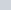,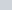andhaving position vector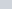,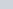andrespectively from the origin as shown in following figure.

If we bring chargefirst from infinity to positionthen there is no work done required to do so, it is because when we bring chargefrom infinity to positionthen at that position there is no any source which can produce electric field. If there is no electric field then there is no any opposing force. Hence work done is zero.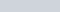But when we bring chargefrom infinity to the positionthen in this, we have to do work done because, here a opposing field is present due to charge. So we have to do work done in against the electric field produced by the first electric charge.
The work done in bringing chargefrom infinity to the positionis-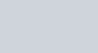Where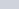is the position vector between chargeandand V is the electric potential due to chargeat position vector.
Now, chargesandwill produce a electric potential at any point say P. Think that point P denotes the position of charge. The position vector between chargeandwill beand in between chargeandwill be.

Now the electric potential due to the chargeandat point P is given as-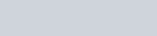So the work done in bringing chargefrom infinity to the positionis-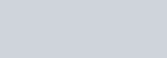The total work done in assembling the charges at the given location is equal the total potential energy of the system and According to the superposition principle, this total potential energy can be obtained by adding the work done of individual charges.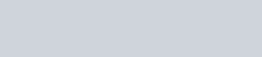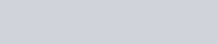This result can also be expressed in the form of summation as follows-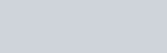If we want to obtain the value of electric potential energy of a system of N point charges then we also obtained it. The value of electric potential energy due to a system of N point charges is equal to the total amount of work done in assembling all the charges to the given position from infinity.Watch this video for more reference:

We know that electrostatic force is conservative in nature, so the value of U is independent of the manner in which the configuration of charge is assembled.
The SI unit of electric potential energy is joule (J) and it’s another unit is electron volt (eV)